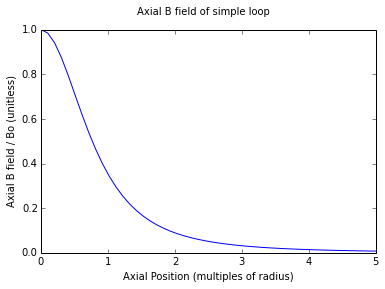# On-Axis Field Due to a Current Loop¶

This simple formula uses the Law of Biot Savart, integrated over a circular current loop to obtain the magnetic field at any point along the axis of the loop.$B = \frac {\mu_o i r^2}{2(r^2 + x^2)^{\frac 3 2}}$

B is the magnetic field, in teslas, at any point on the axis of the current loop. The direction of the field is perpendicular to the plane of the loop.

$\mathbf \mu_o$ is the permeability constant (1.26x10-6 Hm-1)

i is the current in the wire, in amperes.

r is the radius of the current loop, in meters.

x is the distance, on axis, from the center of the current loop to the location where the magnetic field is calculated, in meters.

## Special Case: x = 0¶

$B = \frac {\mu_o i}{2 r}$

## Special Case: x >> 0¶

$B = \frac {\mu_o i r^2}{2 x^3}$

Note that this is equivalent to the expression for on-axis magnetic field due to a magnetic dipole:

$B = \frac {\mu_o i A}{2 \pi x^3}$

where A is the area of the current loop, or $\pi r^2$.

## Code Example¶

The following IPython code illustrates how to compute the on-axis field due to a simple current loop.

In :
%matplotlib inline
from scipy.special import ellipk, ellipe, ellipkm1
from numpy import pi, sqrt, linspace
from pylab import plot, xlabel, ylabel, suptitle, legend, show

uo = 4E-7*pi     # Permeability constant - units of H/m

# On-Axis field = f(current and radius of loop, x of measurement point)
def Baxial(i, a, x, u=uo):
if a == 0:
if x == 0:
return NaN
else:
return 0.0
else:
return (u*i*a**2)/2.0/(a**2 + x**2)**(1.5)


Use the Baxial function to compute the central field of a unit loop (1 meter radius, 1 ampere of current), in teslas:

In :
print("{:.3} T".format(Baxial(1, 1, 0)))

6.28e-07 T


You can try selecting your own current (a), radius (m) and axial position (m) combination to see what the resulting field is:

In :
from ipywidgets import interactive
from IPython.display import display

def B(i, a, x):
return "{:.3} T".format(Baxial(i,a,x))

v = interactive(B, i=(0.0, 20.0), a=(0.0, 10.0), x=(0.0, 10.0))
display(v)

'6.28e-07 T'

Now plot the field intensity, as a fraction of the central field, at various positions along the axis (measured as multiples of the coil radius):

In :
axiallimit = 5.0 # meters from center